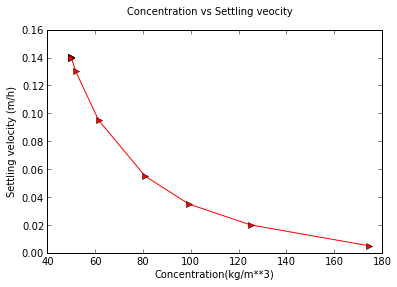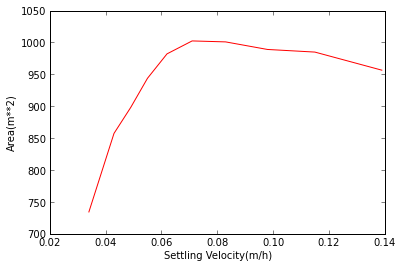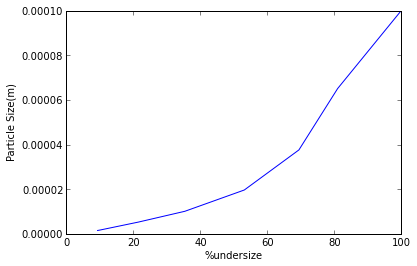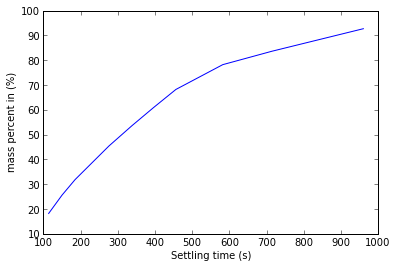# Chapter 10 : SEdimentation and classification¶

### example 10.1 pageno : 186¶

In :
%matplotlib inline
import math
from matplotlib.pyplot import *
#example 10.1
# Initialization of Variable
t = [0, 0.5, 1. ,2. ,3., 4., 5., 6., 7., 8., 9., 10.]             #time
h = [1.10 ,1.03, .96, .82, .68, .54, .42, .35, .31, .28, .27, .27]
Cl = [0 ,0, 0, 0, 0, 0, 0, 0, 0, 0, 0]
m = 0.05
V = 1/1000.             #volume
v = [0,0,0,0,0,0,0,0,0,0,0]

#calculations
Co = m/V            #concentration at t = 0
v = (h-h)/(t-t)
Cl = Co
for i in range(1,11):
v[i] = (h[i-1]-h[i+1])/(t[i+1]-t[i-1])          #slope or settling velocity
Cl[i] = Co*h/(h[i]+v[i]*t[i])

plot(t,h,'r--d')
clf()
plot(Cl,v,'r->')
print Cl,v
suptitle("Concentration vs Settling veocity")
xlabel("Concentration(kg/m**3)")
ylabel("Settling velocity (m/h)")
show()

Populating the interactive namespace from numpy and matplotlib
[50.0, 50.0, 50.0, 50.000000000000014, 50.000000000000014, 51.88679245283019, 61.452513966480446, 80.88235294117649, 99.09909909909915, 125.00000000000003, 174.60317460317458] [0.14000000000000012, 0.14000000000000012, 0.14000000000000004, 0.13999999999999996, 0.13999999999999996, 0.13000000000000003, 0.09500000000000003, 0.05499999999999999, 0.034999999999999976, 0.01999999999999999, 0.0050000000000000044]

WARNING: pylab import has clobbered these variables: ['draw_if_interactive']
%pylab --no-import-all prevents importing * from pylab and numpy### example 10.2 page no : 188¶

In :
import math
from numpy import linspace
from matplotlib.pyplot import *

# Initialization of Variable
t = [0, 0.5, 1 ,2, 3, 4, 5, 6, 7, 8, 9, 10]         #time
h = [1.10 ,1.03, .96, .82, .68, .54, .42, .35, .31, .28, .27, .27]
Cl = linspace(50,100,5)
U = [19.53, 17.71, 16.20, 14.92, 13.82, 12.87, 12.04, 11.31, 10.65, 9.55]           #mass ratio of liquid to solid
v = [0.139, 0.115, 0.098, 0.083, 0.071, 0.062, 0.055, 0.049, 0.043, 0.034]                   #terminal velocity

#above value taken from graph given with ques.
C = 130.            #conc. of solids
Q = 0.06            #slurry rate
Cmax = 130.         #maximum solid conc.
rhos = 2300.        #density of solid
rho = 998.          #density of water
V = rho*(1/C-1/rhos)
F = Q*Cl*3600.
A = [0,0,0,0,0,0,0,0,0,0]
for i in range(10):
A[i] = F*(U[i]-V)/rho/v[i]

plot(v,A,'r-')
xlabel("Settling Velocity(m/h)")
ylabel("Area(m**2)")
show()

#maxima finding using datatraveller in the graph
print "the area for each settling velocity",A
print "1005 m**2 is the maximum area found out from the plot"

Qu = Q-F/3600./Cmax
print  "Volumetric flow rate of clarified water in (m**3/s): %.4f"%Quthe area for each settling velocity [956.58577326448562, 984.95679741043818, 989.07500346402981, 1000.9355052127868, 1002.4484410905754, 982.14964830945723, 943.84207758782964, 898.19417690065814, 857.42395579055744, 734.27713187539746]
1005 m**2 is the maximum area found out from the plot
Volumetric flow rate of clarified water in (m**3/s): 0.0369


### example 10.3 pageno : 192¶

In :
import math
from numpy import linspace

# Initialization of Variable
rho1 = 2600.        #density lighter
rho2 = 5100.        #density heavier
pd1 = linspace(0.000015,0.000095,9)          #particle diameter lighter
pd2 = linspace(0.000025,0.000095,8)           #particle diameter heavier
wp1 = [0 ,22 ,35, 47, 59, 68, 75, 81 ,100]                  #weight distribution lighter
wp2 = [0, 21, 33.5, 48, 57.5, 67 ,75, 100]                 #weight distribution heavier
rho = 998.6                                         #density water
mu = 1.03/1000                                      #viscosity water
g = 9.81
u = 0.004                                           #velocity of water
d = 95/1000000.                                     #paeticle diameter maximum

#calculation
#part 1
Re = d*u*rho/mu
d1 = math.sqrt(18*mu*u/g/(rho1-rho))
d2 = math.sqrt(18*mu*u/g/(rho2-rho))
def inter(d,f,g,b):                                 #interpolation linear
for i in range(b):
if d <= f[i+1] and d>f[i]:
break
else:
continue
a = (d-f[i])/(f[i+1]-f[i])*(g[i+1]-g[i])+g[i]
return a

a = inter(d1,pd1,wp1,9)
b = inter(d2,pd2,wp2,8)
v2 = 1./(1+5.)*100.-b/100.*1./(1+5)*100
v1 = 5./(1+5.)*100.-a/100.*5./(1+5)*100
pl2 = (v2)/(v2+v1)
print  "The fraction of heavy ore remained in bottom %.4f"%pl2

#part 2
rho = 1500.
mu = 6.25/10000
a = math.log10(2*d**3*rho*g*(rho1-rho)*3*mu**2)     #math.log10(Re**2(R/rho/mu**2))

#using value from chart(graph)
Re = 10.**0.2136
u = Re*mu/rho/d
d2 = math.sqrt(18*mu*u/g/(rho1-rho))
b = inter(d2,pd2,wp2,8)
print "The percentage of heavy ore left in this case %.4f"%(100-b+3.5)

#part 3
a = 0.75                                        #% of heavy ore in overhead product
s = 100.*5./6./(100*5./6+0.75*100./6)
print "the fraction of light ore in overhead product: %.4f"%s

#part 4
da = pd2
db = pd1
rho = (da**2*rho2-db**2*rho1)/(-db**2+da**2)
print "The minimum density required to seperate 2 ores in kg/m**3: %.4f"%rho

The fraction of heavy ore remained in bottom 0.3197
The percentage of heavy ore left in this case 24.8188
the fraction of light ore in overhead product: 0.8696
The minimum density required to seperate 2 ores in kg/m**3: 2413.9881


### example 10.4 page no : 198¶

In :
import math
from numpy import true_divide

# Initialization of Variable

rho = 998.
w0 = 40.            #density of slurry
mu = 1.01/1000
g = 9.81
rho1 = 2660.        #density quartz
h = 0.25
t = 18.5*60
mp = [5 ,11.8, 20.2, 24.2, 28.5, 37.6 ,61.8]
d = true_divide([30.2, 21.4, 17.4, 16.2, 15.2, 12.3, 8.8],1000000)
u = h/t
d1 = math.sqrt(18*mu*u/g/(rho1-rho))
def inter(d,f,g,b):            #interpolation linear
for i in range(b):
if d > f[i+1] and d <= f[i]:
break
else:
continue
break

a = -(d-f[i+1])/(f[i]-f[i+1])*(g[i+1]-g[i])+g[i+1]
return a
a = inter(d1,d,mp,6)
phi = 1-a/100.
rhot = phi*(rho1-rho)/rho1*w0+rho
print "the density of suspension at depth 25cm in kg/m**3 is %.4f"%rhot

the density of suspension at depth 25cm in kg/m**3 is 1016.5653


### example 10.5 pag eno : 200¶

In :
import math
from matplotlib.pyplot import *

# Initialization of Variable
t = [0, 45, 135, 495, 1875, 6900, 66600, 86400]             #time
m = [0.1911, 0.1586, 0.1388, 0.1109, 0.0805, 0.0568, 0.0372, 0.0359]        #mass total
rho1 = 3100.                    #density of cement
mu = 1.2/1000                   #viscosity of desperant liquid
rho = 790.                      #density of desperant liquid
h = 0.2
V = 10.
s = 0.
g = 9.81
d = [0,0,0,0,0,0,0,0]
mc = [0,0,0,0,0,0,0,0]
mp = [0,0,0,0,0,0,0,0]
d = 100./1000000             #assumed value

for i in range(7):
d[i+1] = math.sqrt(18*mu*h/g/t[i+1]/(rho1-rho))         #dia of particles
mc[i+1] = m[i+1]-0.2/100*V                              #mass of cement
s = s+mc[i+1]

mc = m-0.2*V/100
s = s+mc
mp = 100.

for i in range(7):
mp[i+1] = mc[i+1]/mc*100.            #mass percent below size

plot(mp,d)
xlabel("%undersize")
ylabel("Particle Size(m)")

show()
u = h/t
Re = d*u*rho/mu
if Re<2:
print "since Re<2 for 81% of particles so settlement occurs mainly by stoke-s law"since Re<2 for 81% of particles so settlement occurs mainly by stoke-s law


### example 10.6 page no : 204¶

In :
import math
from matplotlib.pyplot import *

# Initialization of Variable
rho = 998.
rho1 = 2398.            #density of ore
mu = 1.01/1000.
g = 9.81
h = 25/100.
t = [114. ,150., 185., 276., 338., 396., 456., 582., 714., 960.]
m = [0.1429 ,0.2010, 0.2500, 0.3564, 0.4208, 0.4781, 0.5354 ,0.6139, 0.6563, 0.7277]
d = [0,0,0,0,0,0,0,0,0,0]
P = [0,0,0,0,0,0,0,0,0,0]

for i in range(10):
ms = 0.0573+m        #total mass setteled
d[i] = math.sqrt(18.*mu*h/g/(rho1-rho)/t[i])
P[i] = m[i]/ms*100      #mass percent of sample

plot(t,P)
xlabel("Settling time (s)")
ylabel("mass percent in (%)")
show()
print "& its percentage mass distribution respectively" ,"the particle size distribution in (m)" ,P,d
W = [0,0,0,0,0,0,0,0,0,0]
de = [0,0,0,0,0,0,0,0,0,0]
for i in range(9):
de[i] = (P[i+1]-P[i-1])/(t[i+1]-t[i-1])        #slope
W[i] = P[i]-t[i]*de[i]
W = P-P

W = P-t * 0.025
print "mass and diameter(m)respectively with serial no:"

for i in range(4,10):
print i-4,
print "mass, is",
print "for diameter in(m) of %f   %f"%(W[i],d[i])& its percentage mass distribution respectively the particle size distribution in (m) [18.203821656050955, 25.605095541401273, 31.84713375796178, 45.40127388535032, 53.60509554140127, 60.904458598726116, 68.20382165605096, 78.20382165605095, 83.60509554140127, 92.70063694267515] [5.387856206749596e-05, 4.6970241455099756e-05, 4.229436978392301e-05, 3.4626921406038745e-05, 3.129032486308103e-05, 2.890818526183746e-05, 2.693928103374798e-05, 2.3845527268417837e-05, 2.1528774873471063e-05, 1.856661815577208e-05]
mass and diameter(m)respectively with serial no:
0 mass, is for diameter in(m) of 9.937792   0.000031
1 mass, is for diameter in(m) of 11.912124   0.000029
2 mass, is for diameter in(m) of 25.792480   0.000027
3 mass, is for diameter in(m) of 43.461413   0.000024
4 mass, is for diameter in(m) of 56.222222   0.000022
5 mass, is for diameter in(m) of 68.700637   0.000019


### example 10.7 page no : 208¶

In :
import math

# Initialization of Variable

rho = 1002.             #density of print erant
rho1 = 2240.            #density of kaolin
mu = 1.01/1000          #viscosity
g = 9.81
t = 600.
h2 = 0.2
h1 = 0.4
dg = 15.*10**-6         #particle size to be removed

#calculations
#part 1
d = math.sqrt(18*mu*h2/g/(rho1-rho)/t)
x = dg/d
f = h2/h1*(1-x**2)      #fraction separated after first decanting
g = f*(1-f)
print "fraction of particles separated after second decanting %.4f"%g
print "total fraction of particles separated after decanting %.4f"%(f+g)

#part 2
h = (1.-20/40.*(1-x**2))**6
print "fraction of particles separated after sixth decanting %.4f"%h

fraction of particles separated after second decanting 0.1992
total fraction of particles separated after decanting 0.4737
fraction of particles separated after sixth decanting 0.1458

In [ ]: